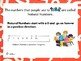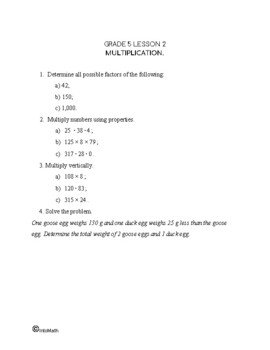CA\$2.00

We use numbers everyday  to count, to measure, to order and estimate and so on.

Natural numbers are positive numbers that we use to count and order.

0, 3, 6, 10, 204, 1000…

In mathematical terminology, numbers used for counting are called “cardinal numbers” and numbers used for ordering are called “ordinal numbers”.

Mathematicians use N to represent a set of all natural numbers. The set of natural numbers is an infinite set. This infinity is called countable infinity.CA\$4.00

Properties of natural numbers:

1. 0 is a natural number (but some mathematicians do not include it in the list of natural numbers)

2. Every natural number has a number that comes after it and is also a natural number

3. 0 does not come after any natural number

4. If the number that comes after x is equal to the number that comes after y, then x = y

### Operations with numbers up to 1000

We can do several operations with natural numbers: order, compare, add, subtract, multiply and divide them.

In this lesson you will learn how to add and subtract natural numbers up to 1000 by breaking the numbers down based on their place value. Place value of a digit is a very important concept.

For example, 345 could be represented as a sum of 300 + 40 + 5. When adding it to another similar number, it often makes sense to add the components separately.

Subtracting two natural numbers does not always result in a natural number. For example, 213 – 321 will produce a negative number, that is no longer a member of a set of natural numbers, but rather a negative integer.

Place value of digits within a number is important when doing operations with natural numbers, as corresponding digits need to be added/subtracted respectively.